Updating search results...

# 10 Results

View
Selected filters:
• MCCRS.Math.Content.8.NS.A.2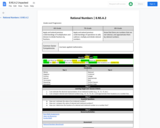Conditional Remix & Share Permitted
CC BY-NC-SA
Rating
0.0 stars

Estimate the value and compare the size of irrational numbers and approximate their locations on a number line.

Subject:
Mathematics
Material Type:
Activity/Lab
Author:
Liberty Public Schools
04/13/2021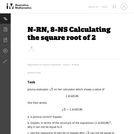Unrestricted Use
CC BY
Rating
0.0 stars

This task is intended for instructional purposes so that students can become familiar and confident with using a calculator and understanding what it can and cannot do. This task gives an opportunity to work on the notion of place value (in parts (b) and (c)) and also to understand part of an argument for why the square root of two is not a rational number.

Subject:
Mathematics
Material Type:
Activity/Lab
Provider:
Illustrative Mathematics
Provider Set:
Illustrative Mathematics
Author:
Illustrative Mathematics
11/13/2012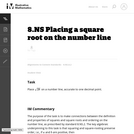Unrestricted Use
CC BY
Rating
0.0 stars

This is a task from the Illustrative Mathematics website that is one part of a complete illustration of the standard to which it is aligned. Each task has at least one solution and some commentary that addresses important asects of the task and its potential use. Here are the first few lines of the commentary for this task: Place $\sqrt{28}$ on a number line, accurate to one decimal point....

Subject:
Mathematics
Material Type:
Activity/Lab
Provider:
Illustrative Mathematics
Provider Set:
Illustrative Mathematics
Author:
Illustrative Mathematics
05/29/2013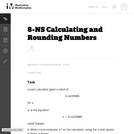Unrestricted Use
CC BY
Rating
0.0 stars

This task is intended for instructional (rather than assessment) purposes, providing an opportunity to discuss technology as it relates to irrational numbers and calculations in general. The task gives a concrete example where rounding and then multiplying does not yield the same answer as multiplying and then rounding.

Subject:
Mathematics
Material Type:
Activity/Lab
Provider:
Illustrative Mathematics
Provider Set:
Illustrative Mathematics
Author:
Illustrative Mathematics
09/13/2012Unrestricted Use
CC BY
Rating
0.0 stars

This task can be used to either build or assess initial understandings related to rational approximations of irrational numbers.

Subject:
Mathematics
Material Type:
Activity/Lab
Provider:
Illustrative Mathematics
Provider Set:
Illustrative Mathematics
Author:
Illustrative Mathematics
05/01/2012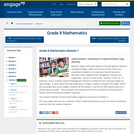Conditional Remix & Share Permitted
CC BY-NC-SA
Rating
0.0 stars

Module 7 begins with work related to the Pythagorean Theorem and right triangles.  Before the lessons of this module are presented to students, it is important that the lessons in Modules 2 and 3 related to the Pythagorean Theorem are taught (M2:  Lessons 15 and 16, M3:  Lessons 13 and 14).  In Modules 2 and 3, students used the Pythagorean Theorem to determine the unknown length of a right triangle.  In cases where the side length was an integer, students computed the length.  When the side length was not an integer, students left the answer in the form of x2=c, where c was not a perfect square number.  Those solutions are revisited and are the motivation for learning about square roots and irrational numbers in general.

Subject:
Geometry
Mathematics
Material Type:
Module
Provider:
New York State Education Department
Provider Set:
EngageNY
02/02/2014Unrestricted Use
CC BY
Rating
0.0 stars

My goal is to merge New York State standards with Common Core Standards and Integrated Algebra Regent Standards for our 8th grade curriculum.

Subject:
Mathematics
Material Type:
Full Course
Lesson Plan
Syllabus
01/28/2016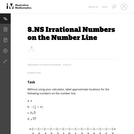Unrestricted Use
CC BY
Rating
0.0 stars

When students plot irrational numbers on the number line, it helps reinforce the idea that they fit into a number system that includes the more familiar integer and rational numbers.

Subject:
Mathematics
Material Type:
Activity/Lab
Provider:
Illustrative Mathematics
Provider Set:
Illustrative Mathematics
Author:
Illustrative Mathematics
05/01/2012Conditional Remix & Share Permitted
CC BY-NC
Rating
0.0 stars

Four full-year digital course, built from the ground up and fully-aligned to the Common Core State Standards, for 7th grade Mathematics. Created using research-based approaches to teaching and learning, the Open Access Common Core Course for Mathematics&nbsp;is designed with student-centered learning in mind, including activities for students to develop valuable 21st century skills and academic mindset.

Subject:
Mathematics
Material Type:
Full Course
Provider:
Pearson
10/06/2016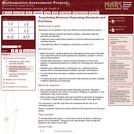Only Sharing Permitted
CC BY-NC-ND
Rating
0.0 stars

This lesson unit is intended to help teachers assess how well students are able to: translate between decimal and fraction notation, particularly when the decimals are repeating; create and solve simple linear equations to find the fractional equivalent of a repeating decimal; and understand the effect of multiplying a decimal by a power of 10.

Subject:
Algebra
Mathematics
Ratios and Proportions
Material Type:
Assessment
Lesson Plan
Provider:
Shell Center for Mathematical Education
Provider Set:
Mathematics Assessment Project (MAP)